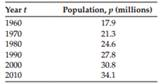### Create an Account

Home / Questions / The data in the following table shows the population of Canada recorded every 10 years bet...

# The data in the following table shows the population of Canada recorded every 10 years between 1960 and 2010. a Find the rate of population growth in 2010 using the three-point backward difference

The data in the following table shows the population of Canada recorded every 10 years between 1960 and 2010.

a. Find the rate of population growth in 2010 using the three-point backward difference formula,

b. Using the result of (a), and applying the two-point central difference formula, predict the population in 2020.Jul 22 2020 View more View LessSubscribe To Get Solution